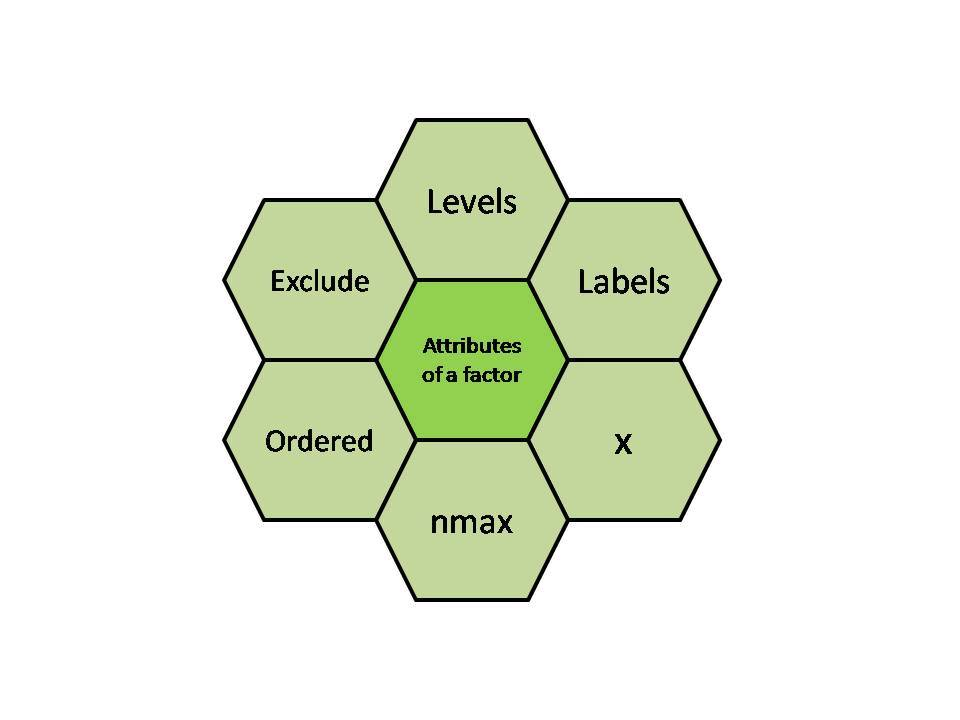# R – Factors

Factors are data structures which are implemented to categorize the data or represent categorical data and store it on multiple levels.
They can be stored as integers with a corresponding label to every unique integer. Though factors may look similar to character vectors, they are integers and care must be taken while using them as strings.
The factor accepts only a restricted number of distinct values. For example, a data field such as gender may contain values only from female, male or transgender.

In the above example, all the possible cases are known beforehand and are predefined. These distinct values are known as levels. After a factor is created it only consists of levels that are by default sorted alphabetically.

#### Attributes of a Factor

• x: It is the vector which needs to be converted into a factor.
• Levels: It is a set of distinct values which are given to the input vector x.
• Labels: It is a character vector corresponding to the number of labels.
• Exclude: This will mention all the values you want to exclude.
• Ordered: This logical attribute decide whether the levels are ordered.
• nmax: It will decide the upper limit for the maximum number of levels.#### Creating a Factor

The command used to create or modify a factor in R language is – `factor()` with a vector as input.
The two steps to creating a factor are:

• Creating a vector
• Converting the vector created into a factor using function factor()

Examples: Let us create a factor gender with levels female, male and transgender.

 `# Creating a vector ` `x<``-``c(``"female"``, ``"male"``, ``"male"``, ``"female"``) ` `print``(x) ` ` `  `# Converting the vector x into a factor named gender ` `gender<``-``factor(x) ` `print``(gender) `

Output:

``` "female" "male"   "male"   "female"
 female male   male   female
Levels: female male
```

Levels can also be predefined by the programmer.

 `# Creating a factor with levels defined by programmer ` `gender <``-` `factor(c(``"female"``, ``"male"``, ``"male"``, ``"female"``), ` `          ``levels ``=` `c(``"female"``, ``"transgender"``, ``"male"``)); ` `gender `

Output:

``` female male   male   female
Levels: female transgender male
```

Further one can check the levels of a factor by using function `levels()`.

#### Checking for a Factor

Function`is.factor()` is used to check whether the variable is a factor and returns “TRUE” if it is a factor.

 `gender <``-` `factor(c(``"female"``, ``"male"``, ``"male"``, ``"female"``)); ` `print``(``is``.factor(gender)) `

Output:

` TRUE`

Function `class()` is also used to check whether the variable is a factor and if true returns “factor”.

 `gender <``-` `factor(c(``"female"``, ``"male"``, ``"male"``, ``"female"``)); ` `class``(gender) `

Output:

` "factor"`

#### Accessing elements of a Factor

Like we acess elements of a vector, same way we acess the elements of a factor. If gender is a factor then gender[i] would mean acessing i th element in the factor.
Example:

 `gender <``-` `factor(c(``"female"``, ``"male"``, ``"male"``, ``"female"``)); ` `gender[``3``] `

Output:

``` male
Levels: female male
```

More than one element can be accessed at a time.
Example:

 `gender <``-` `factor(c(``"female"``, ``"male"``, ``"male"``, ``"female"``)); ` `gender[c(``2``, ``4``)] `

Output:

``` male   female
Levels: female male
```

Example:

 `gender <``-` `factor(c(``"female"``, ``"male"``, ``"male"``, ``"female"`  `)); ` `gender[``-``3``] `

Output:

``` female male   female
Levels: female male
```

#### Modification of a Factor

After a factor is formed, its components can be modified but the new values which needs to be assigned must be in the predefined level.

Example:

 `gender <``-` `factor(c(``"female"``, ``"male"``, ``"male"``, ``"female"`  `)); ` `gender[``2``]<``-``"female"` `gender `

Output:

``` female female male   female
Levels: female male
```

For selecting all the elements of the factor gender except ith element, gender[-i] should be used.
So if you want to modify a factor and add value out of predefines levels, then first modify levels.
Example:

 `gender <``-` `factor(c(``"female"``, ``"male"``, ``"male"``, ``"female"`  `)); ` ` `  `# add new level ` `levels(gender) <``-` `c(levels(gender), ``"other"``)     ` `gender[``3``] <``-` `"other"` `gender `

Output:

``` female male   other  female
Levels: female male other
```

#### Factors in Data Frame

The Data frame is similar to a 2D array with the columns containing all the values of one variable and the rows having one set of values from every column. There are four things to remember about data frames:

• column names are compulsory and cannit be empty.
• Unique names should be assigned to each row.
• The data frame’s data can be only of three types- factor, numeric and character type.
• The same number of data items must be present in each column.

In R language when we create a data frame, its column is a categorical data and hence a factor is automatically created on it.

We can create a data frame and check if its column is a factor.
Example:

 `age <``-` `c(``40``, ``49``, ``48``, ``40``, ``67``, ``52``, ``53``)   ` `salary <``-` `c(``103200``, ``106200``, ``150200``, ` `            ``10606``, ``10390``, ``14070``, ``10220``) ` `gender <``-` `c(``"male"``, ``"male"``, ``"transgender"``,  ` `            ``"female"``, ``"male"``, ``"female"``, ``"transgender"``) ` `employee<``-` `data.frame(age, salary, gender)   ` `print``(employee)   ` `print``(``is``.factor(employee\$gender))  `

Output:

```  age salary      gender
1  40 103200        male
2  49 106200        male
3  48 150200 transgender
4  40  10606      female
5  67  10390        male
6  52  14070      female
7  53  10220 transgender
 TRUE
```

My Personal Notes arrow_drop_upCheck out this Author's contributed articles.

If you like GeeksforGeeks and would like to contribute, you can also write an article using contribute.geeksforgeeks.org or mail your article to contribute@geeksforgeeks.org. See your article appearing on the GeeksforGeeks main page and help other Geeks.

Please Improve this article if you find anything incorrect by clicking on the "Improve Article" button below.

Article Tags :

1

Please write to us at contribute@geeksforgeeks.org to report any issue with the above content.